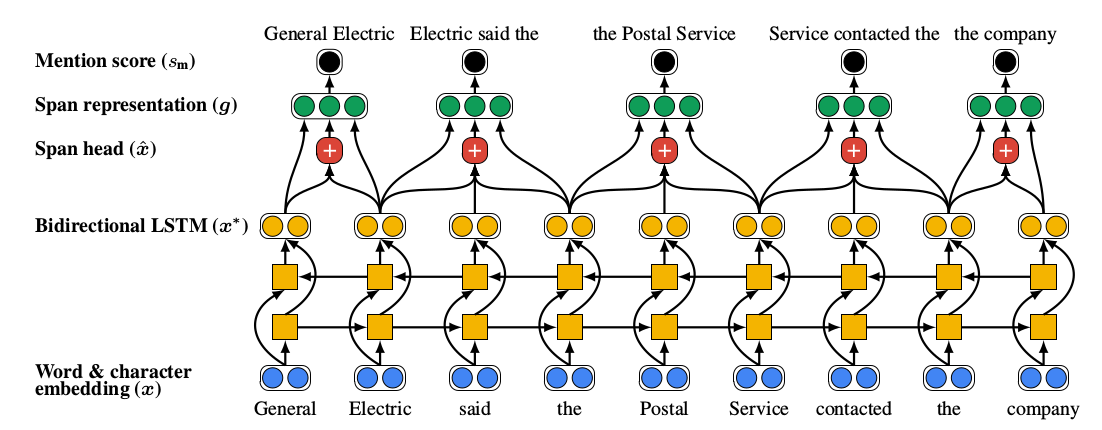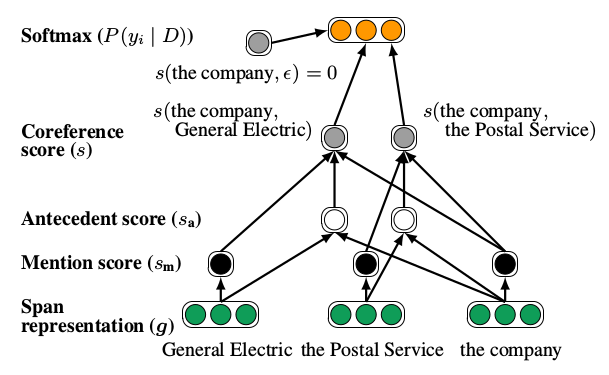# 【转载】指代消解笔记

## 端到端的神经共指消解

Lee K, He L, Lewis M, et al. End-to-end Neural Coreference Resolution[J]. 2017:188-197.

$D$中可能的span的数量$N=\frac{T(T+1)}{2}$。分别用START(i) 和END(i)来表示$D$中span $i$ ($1\leqslant i \leqslant N$)开始和结束时的索引。文中以START(i) 来对span进行排序编号，对于START(i) 相同的span，考虑END(i)。

### 模型部分：

$f(y_1,{\ldots},y_N|D)= \prod_{i=1}^N P(y_i|D) =\prod_{i=1}^N \frac{exp(s(i,y_i))}{\sum_{y' \in \mathcal Y(i)}exp(s(i,y'))}$

$s(i,j)=\begin{cases}0 & j=\epsilon\\s_m(i)+s_m(j)+s_a(i,j) & j\neq \epsilon\end{cases}$Step1：计算每个span的向量表示，并以此对各潜在mention(文本中代表同一实体的不同表述)打分。具体的做法是：将编码信息切分成一组sentence（句子）组，对每一个sentence独立地构建深度学习模型，将特征矩阵输入到深度学习模型（如长短时记忆网络LSTM、卷积神经网络CNN等）中，得到由sentence构成的篇章文本中每一个词的向量表示。对于每个span，将其中的每个词进行组合（如矩阵拼接）得到span的向量表示。然后对span的向量表示进行非线性映射，得到每个潜在mention的分数，并以该分数大小对mention进行修剪，得到一定数量的mention。Step2：对每一对span的向量表示计算先行语得分。通过对两个span的mention score及它们的配对先行语得分求和，得到一对span最终的共指得分。
$s_m(i)=\pmb w_m \cdot {\rm FFNN}_m(\pmb g_i)$
$s_a(i,j)=\pmb w_a \cdot {\rm FFNN}_a([\pmb g_i, \pmb g_j,g_i \circ g_j, \phi (i, j)])$

### 训练过程：

$log\prod_{i=1}^N \sum_{\hat y \in \mathcal Y(i)\cap {\rm GOLD}(i) }P(\hat y)$

（其中，中文的词向量用了预训练好的word2vec）

posted @ 2019-09-30 17:29  致林  阅读(...)  评论(...编辑  收藏